Скачать презентацию CS 332 Algorithms Greedy Algorithms Continued David Luebke

d35d3e80a63570ebe2263e435e9b5610.ppt

• Количество слайдов: 21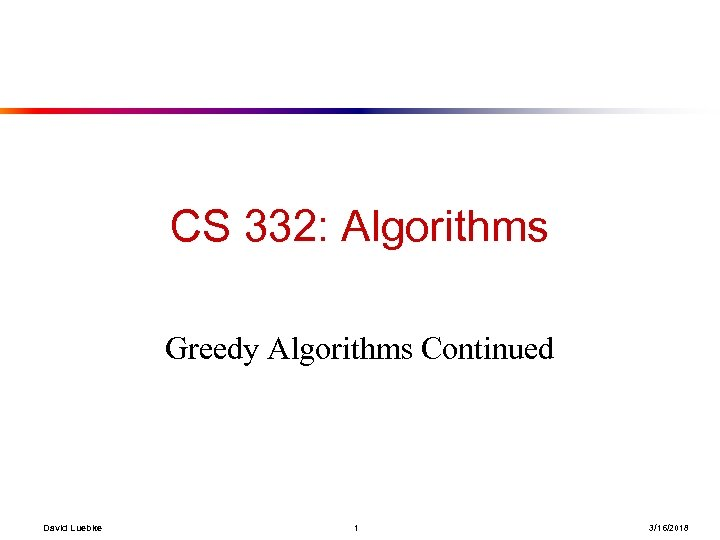CS 332: Algorithms Greedy Algorithms Continued David Luebke 1 3/16/2018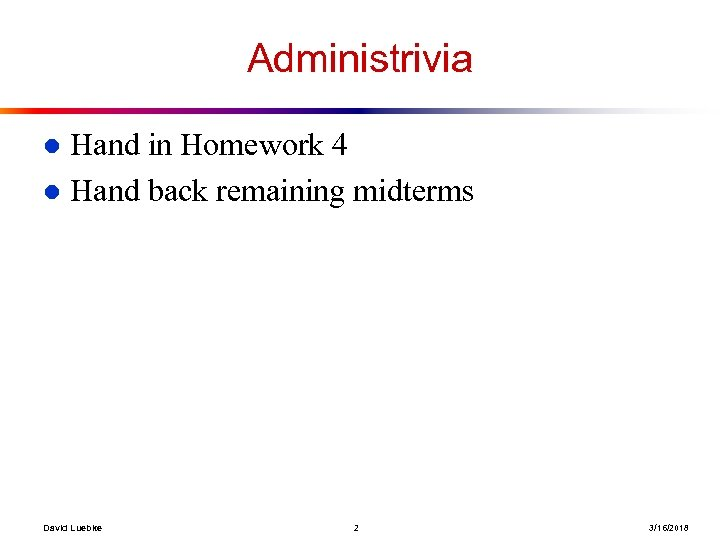Administrivia Hand in Homework 4 l Hand back remaining midterms l David Luebke 2 3/16/2018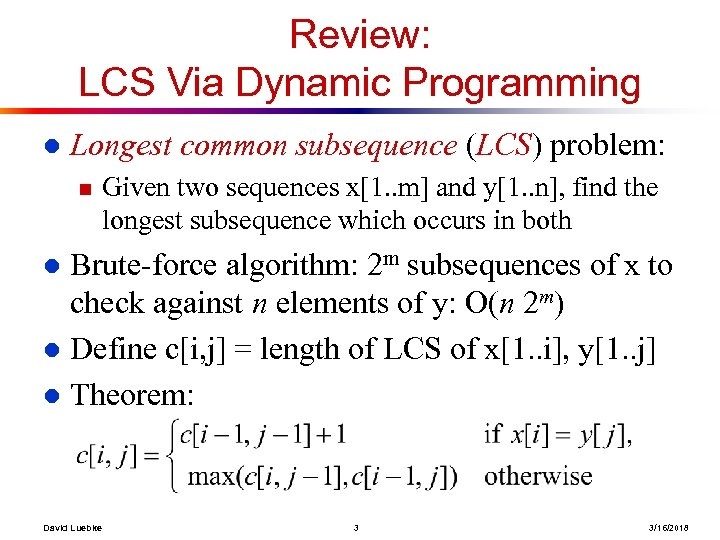Review: LCS Via Dynamic Programming l Longest common subsequence (LCS) problem: n Given two sequences x[1. . m] and y[1. . n], find the longest subsequence which occurs in both Brute-force algorithm: 2 m subsequences of x to check against n elements of y: O(n 2 m) l Define c[i, j] = length of LCS of x[1. . i], y[1. . j] l Theorem: l David Luebke 3 3/16/2018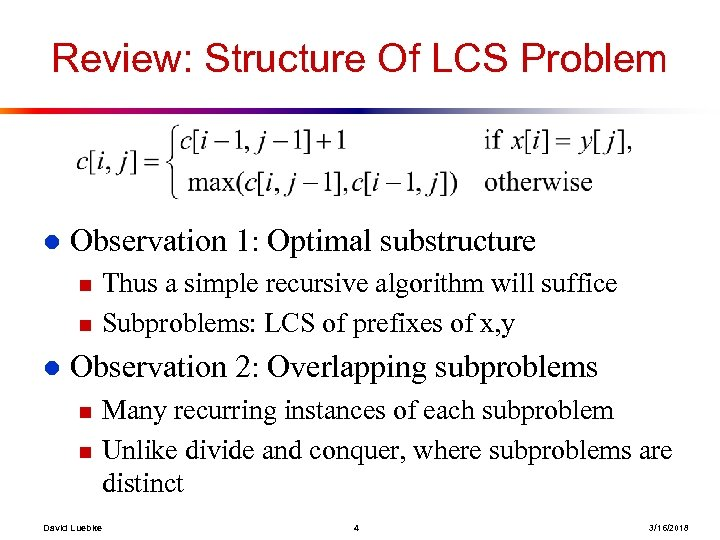Review: Structure Of LCS Problem l Observation 1: Optimal substructure n n l Thus a simple recursive algorithm will suffice Subproblems: LCS of prefixes of x, y Observation 2: Overlapping subproblems n n David Luebke Many recurring instances of each subproblem Unlike divide and conquer, where subproblems are distinct 4 3/16/2018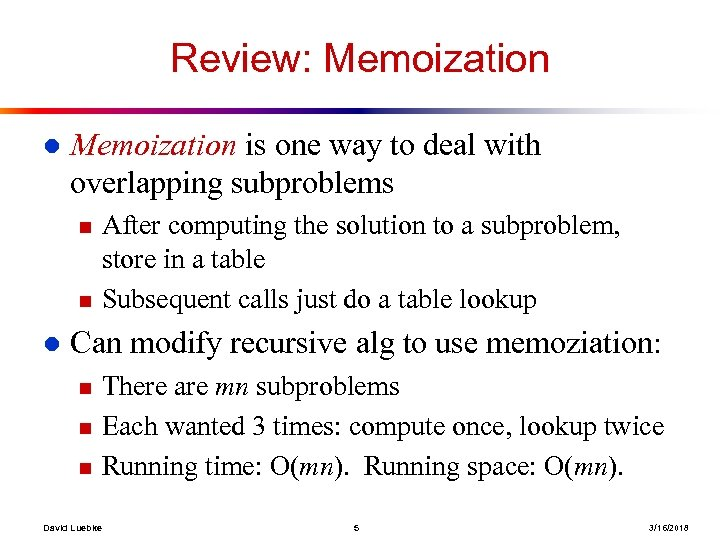Review: Memoization l Memoization is one way to deal with overlapping subproblems n n l After computing the solution to a subproblem, store in a table Subsequent calls just do a table lookup Can modify recursive alg to use memoziation: n n n David Luebke There are mn subproblems Each wanted 3 times: compute once, lookup twice Running time: O(mn). Running space: O(mn). 5 3/16/2018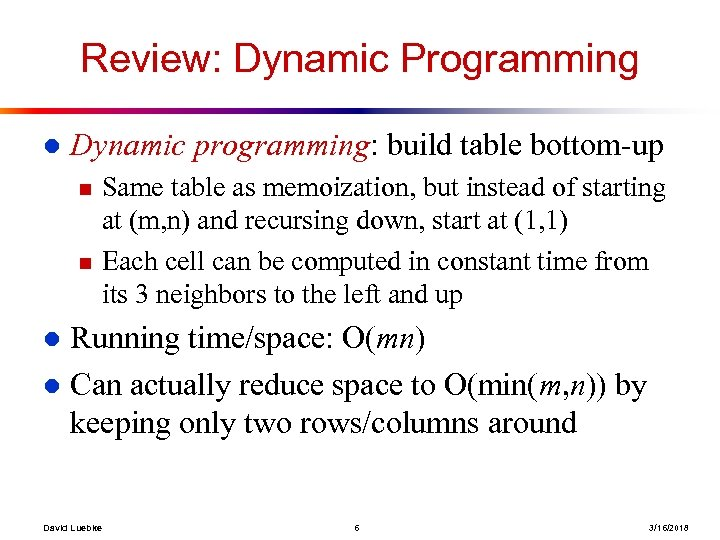Review: Dynamic Programming l Dynamic programming: build table bottom-up n n Same table as memoization, but instead of starting at (m, n) and recursing down, start at (1, 1) Each cell can be computed in constant time from its 3 neighbors to the left and up Running time/space: O(mn) l Can actually reduce space to O(min(m, n)) by keeping only two rows/columns around l David Luebke 6 3/16/2018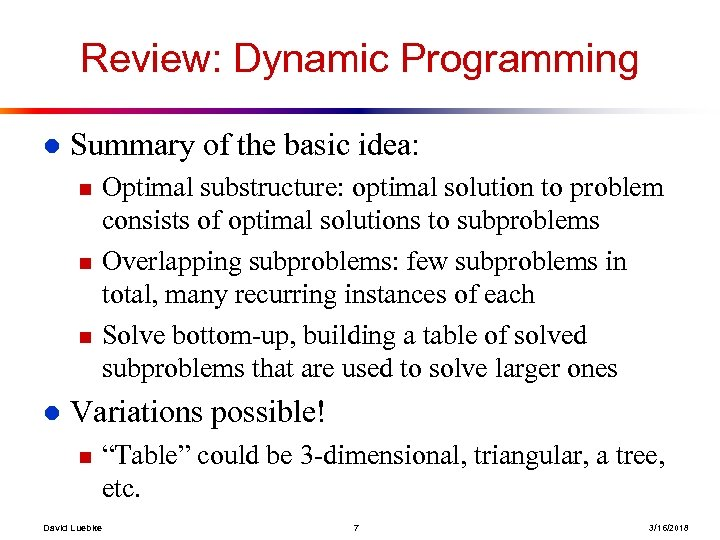Review: Dynamic Programming l Summary of the basic idea: n n n l Optimal substructure: optimal solution to problem consists of optimal solutions to subproblems Overlapping subproblems: few subproblems in total, many recurring instances of each Solve bottom-up, building a table of solved subproblems that are used to solve larger ones Variations possible! n David Luebke “Table” could be 3 -dimensional, triangular, a tree, etc. 7 3/16/2018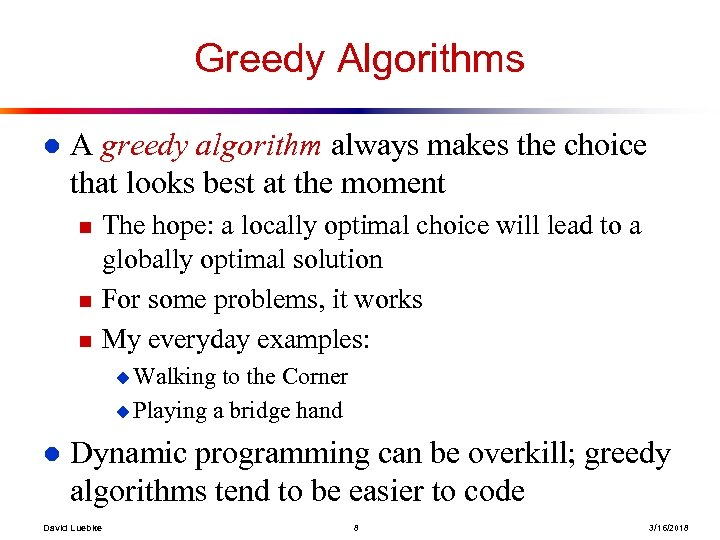Greedy Algorithms l A greedy algorithm always makes the choice that looks best at the moment n n n The hope: a locally optimal choice will lead to a globally optimal solution For some problems, it works My everyday examples: u Walking to the Corner u Playing a bridge hand l Dynamic programming can be overkill; greedy algorithms tend to be easier to code David Luebke 8 3/16/2018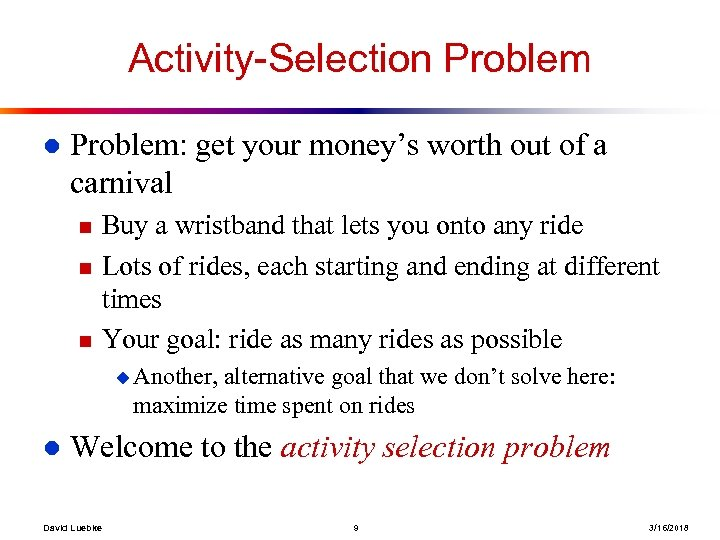Activity-Selection Problem l Problem: get your money’s worth out of a carnival n n n Buy a wristband that lets you onto any ride Lots of rides, each starting and ending at different times Your goal: ride as many rides as possible u Another, alternative goal that we don’t solve here: maximize time spent on rides l Welcome to the activity selection problem David Luebke 9 3/16/2018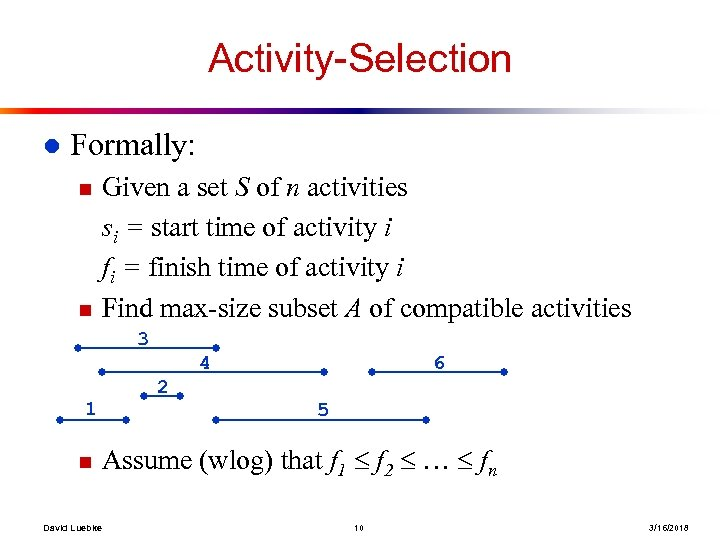Activity-Selection l Formally: n n Given a set S of n activities si = start time of activity i fi = finish time of activity i Find max-size subset A of compatible activities 3 4 1 n David Luebke 6 2 5 Assume (wlog) that f 1 f 2 … fn 10 3/16/2018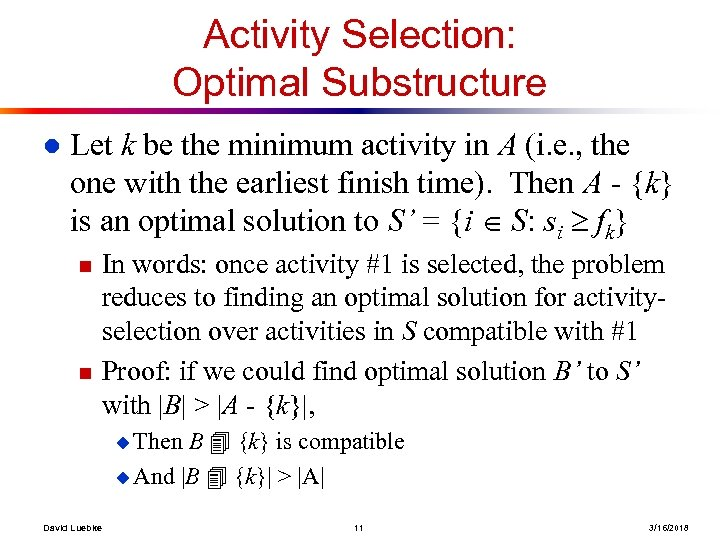Activity Selection: Optimal Substructure l Let k be the minimum activity in A (i. e. , the one with the earliest finish time). Then A - {k} is an optimal solution to S’ = {i S: si fk} n n In words: once activity #1 is selected, the problem reduces to finding an optimal solution for activityselection over activities in S compatible with #1 Proof: if we could find optimal solution B’ to S’ with |B| > |A - {k}|, B {k} is compatible u And |B {k}| > |A| u Then David Luebke 11 3/16/2018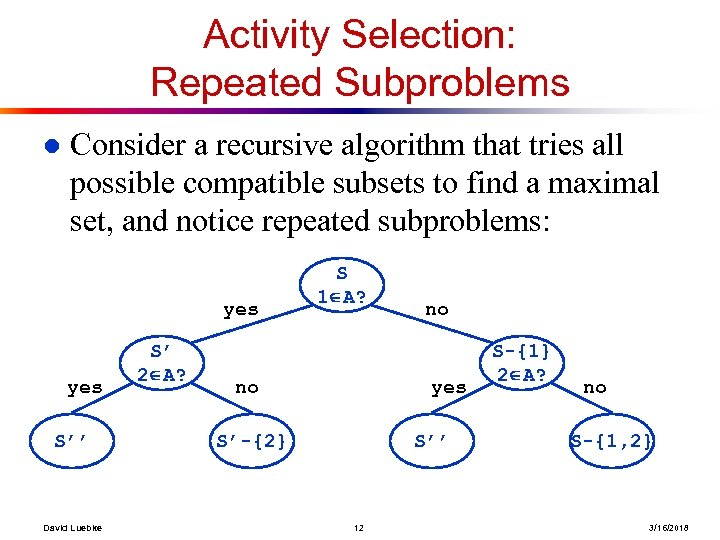Activity Selection: Repeated Subproblems l Consider a recursive algorithm that tries all possible compatible subsets to find a maximal set, and notice repeated subproblems: yes S’’ David Luebke S’ 2 A? S 1 A? no no yes S’-{2} S’’ 12 S-{1} 2 A? no S-{1, 2} 3/16/2018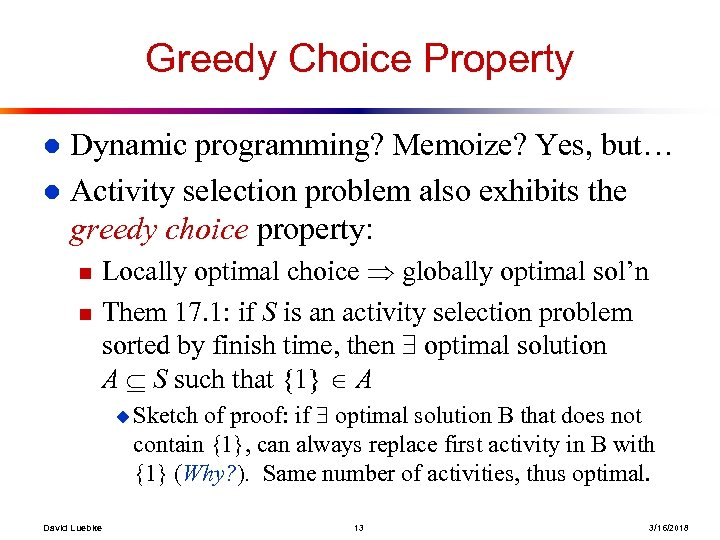Greedy Choice Property Dynamic programming? Memoize? Yes, but… l Activity selection problem also exhibits the greedy choice property: l n n Locally optimal choice globally optimal sol’n Them 17. 1: if S is an activity selection problem sorted by finish time, then optimal solution A S such that {1} A of proof: if optimal solution B that does not contain {1}, can always replace first activity in B with {1} (Why? ). Same number of activities, thus optimal. u Sketch David Luebke 13 3/16/2018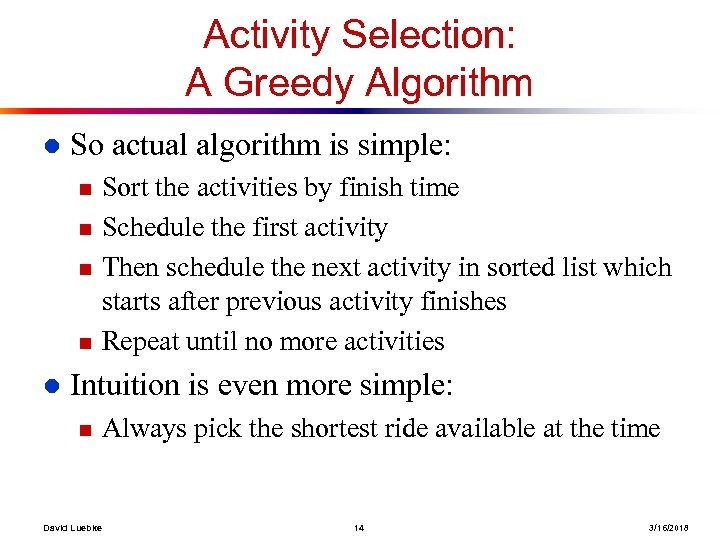Activity Selection: A Greedy Algorithm l So actual algorithm is simple: n n l Sort the activities by finish time Schedule the first activity Then schedule the next activity in sorted list which starts after previous activity finishes Repeat until no more activities Intuition is even more simple: n David Luebke Always pick the shortest ride available at the time 14 3/16/2018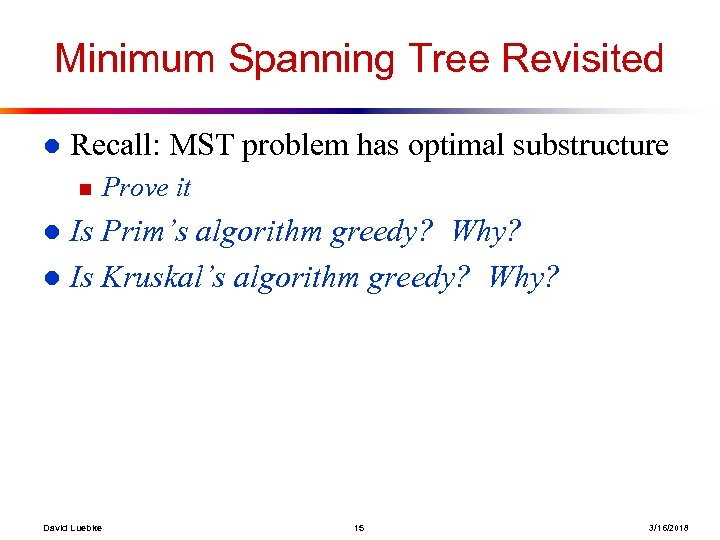Minimum Spanning Tree Revisited l Recall: MST problem has optimal substructure n Prove it Is Prim’s algorithm greedy? Why? l Is Kruskal’s algorithm greedy? Why? l David Luebke 15 3/16/2018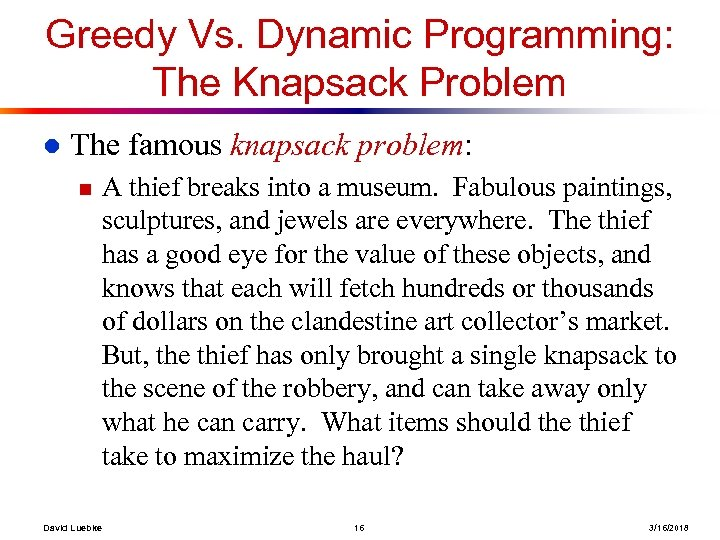Greedy Vs. Dynamic Programming: The Knapsack Problem l The famous knapsack problem: n David Luebke A thief breaks into a museum. Fabulous paintings, sculptures, and jewels are everywhere. The thief has a good eye for the value of these objects, and knows that each will fetch hundreds or thousands of dollars on the clandestine art collector’s market. But, the thief has only brought a single knapsack to the scene of the robbery, and can take away only what he can carry. What items should the thief take to maximize the haul? 16 3/16/2018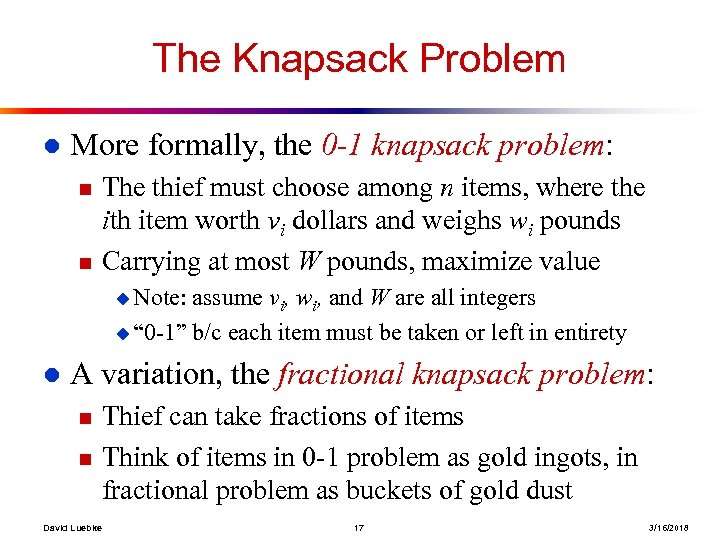The Knapsack Problem l More formally, the 0 -1 knapsack problem: n n The thief must choose among n items, where the ith item worth vi dollars and weighs wi pounds Carrying at most W pounds, maximize value u Note: assume vi, wi, and W are all integers u “ 0 -1” b/c each item must be taken or left in entirety l A variation, the fractional knapsack problem: n n David Luebke Thief can take fractions of items Think of items in 0 -1 problem as gold ingots, in fractional problem as buckets of gold dust 17 3/16/2018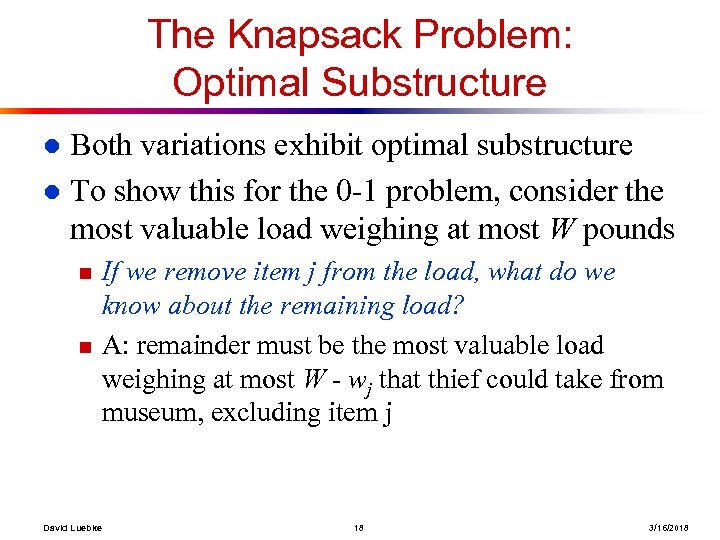The Knapsack Problem: Optimal Substructure Both variations exhibit optimal substructure l To show this for the 0 -1 problem, consider the most valuable load weighing at most W pounds l n n David Luebke If we remove item j from the load, what do we know about the remaining load? A: remainder must be the most valuable load weighing at most W - wj that thief could take from museum, excluding item j 18 3/16/2018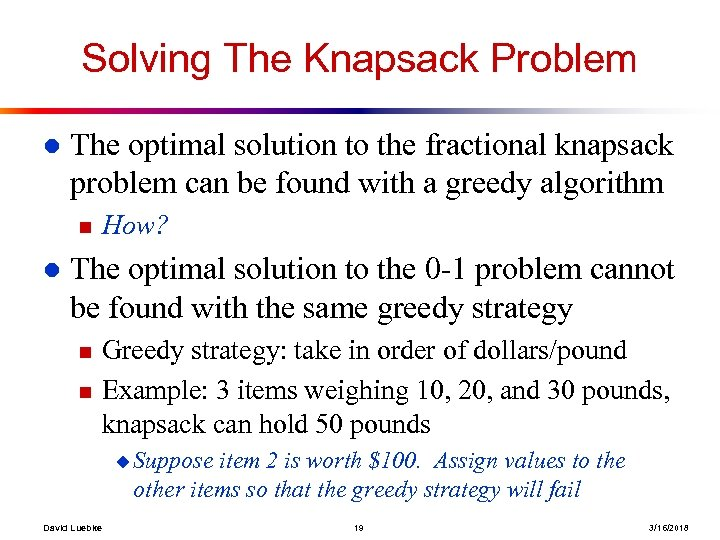Solving The Knapsack Problem l The optimal solution to the fractional knapsack problem can be found with a greedy algorithm n l How? The optimal solution to the 0 -1 problem cannot be found with the same greedy strategy n n Greedy strategy: take in order of dollars/pound Example: 3 items weighing 10, 20, and 30 pounds, knapsack can hold 50 pounds u Suppose item 2 is worth \$100. Assign values to the other items so that the greedy strategy will fail David Luebke 19 3/16/2018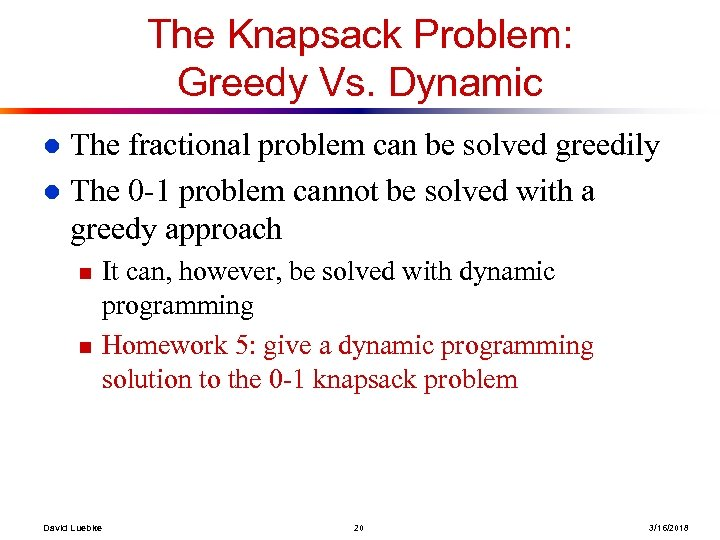The Knapsack Problem: Greedy Vs. Dynamic The fractional problem can be solved greedily l The 0 -1 problem cannot be solved with a greedy approach l n n David Luebke It can, however, be solved with dynamic programming Homework 5: give a dynamic programming solution to the 0 -1 knapsack problem 20 3/16/2018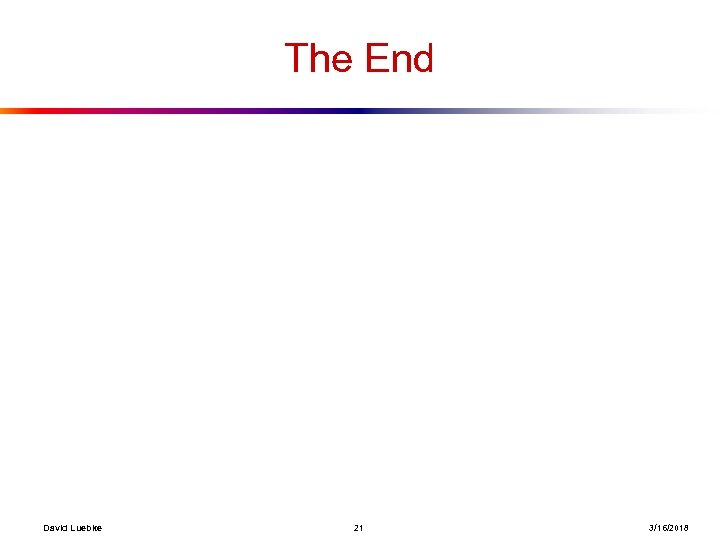The End David Luebke 21 3/16/2018